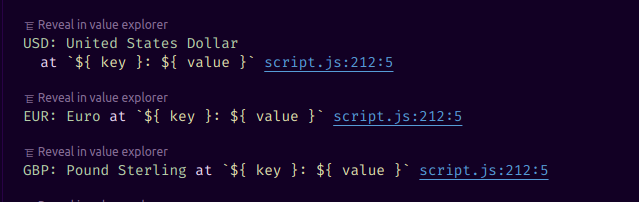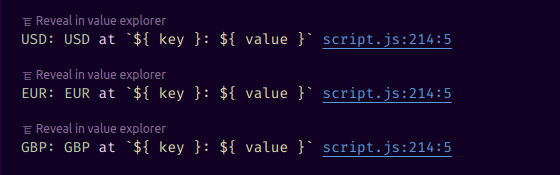# const - JS | lectureWorking With Arrays

forEach With Maps & Sets

In the last lecture, we learned how to use the `forEach` method on arrays. However, `forEach` is also available on maps and sets. Let's take a slight detour now and see how `forEach` works on maps and sets. We are going to start with maps, so let's start by creating a simple currency map.

script.js
``````const currenciesMap = new Map([
['USD', 'United States Dollar'],
['EUR', 'Euro'],
['GBP', 'Pound Sterling'],
]);``````

Now when calling the `forEach` method on the `currenciesMap`, the call back function also has three parameters. The first parameter is the current value, the second parameter is the key, and the third parameter is the map itself.

script.js
``````currenciesMap.forEach(function (value, key, map) {  // do something
});``````

Hence, you can see that it is similar to an array, where the first parameter is the current element of the array, the second parameter is the index of the current element, and the third parameter is the entire array. There is a nice correspondence between these, making it easy to memorize.

Let's now log a nice string here, just to show you that `forEach` works on maps.

script.js
``````currenciesMap.forEach(function (value, key, map) {
console.log(`\${key}: \${value}`);
});``````Let's now try the same with sets.

script.js
``const currenciesSet = new Set(['USD', 'EUR', 'GBP']);``

Let's now call the `forEach` method on the `currenciesSet`.

script.js
``````currenciesSet.forEach(function (value, key, map) {
console.log(`\${key}: \${value}`);
});``````Ah we get something strange here. We get `USD: USD`, `EUR: EUR` and `GBP: GBP`. This means that the key and the value are the same. But why? Well, a set doesn't have keys, and it doesn't have indexes, either. And so there is no value that would make sense for the key. Essentially, the parameter `key` makes no sense at all. It wouldn't even have to be there. Hence, the people who designed the `forEach` method for sets, could have simply omitted the second argument. But if they did that, then the `forEach` method would have been different from the others. And so, that would then create confusion among developers.

It was therefore decided that the signature would remain the same. That is, to keep the same three parameters in the callback function and, simply set an underscore for the second parameter. The underscore simply means a throwaway variable. That is, an unnecessary variable. It's just a convention which we will see again later.

script.js
``````currenciesSet.forEach(function (value, _, map) {  console.log(`\${value}: \${value}`);
});``````